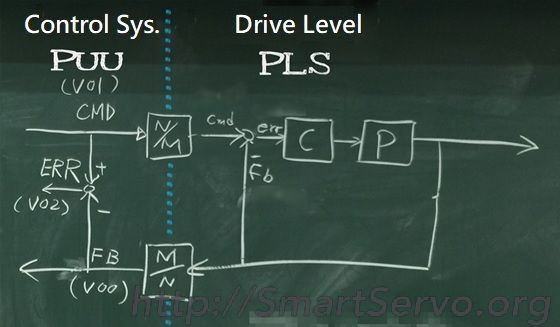# PUU position unit explanation

In the motion control system, there are a number of position counters to record the current position, commands and errors of the machine. It is not appropriate to use PLS as a unit (for reasons see Link). Therefore, a new  position unit must be introduced, called the Position of User Unit (PUU), which is similar to the Pulse Equivalent Unit in the traditional system that uses pulses as the position command , indicates the moving distance of a pulse. Because the current control system can send commands through communication, there is no physical pulse, the user can be more freely choose the desired position unit, ie PUU. The relationship between PUU and PLS is the electronic gear ratio (N/M) defined as follows:

PUU (counts) * N/M (electronic gear ratio numerator/denominator) = PLS (counts)The left side of the electronic gear ratio belongs to the control system and uses PUU units. The right side of the gear ratio belongs to the interior of the drive and uses PLS units [Note 1]. Both sides have position feedback (FB), command (CMD) and error (ERR). And meet ERR = CMD – FB at any time.

[Note 1] by increasing the resolution of the encoder (currently up to 24 bit/Rev), it allows position resolution and low speed Speed ​​estimation is more accurate. The goal of the servo drive is to optimize the performance of the motor control. Naturally, it is better to deal directly with PLS units!

## Key benefits of using PUU units:

1,Easy to observe：Because we are interested in the position of the end of the mechanism, not the position of the motor shaft! Usually the user chooses a common and easy-to-observe unit, such as metric length units (m) or angles (°),as the unit represented by PUU.

• A straight-line mechanism, PUU is usually defined as 10um or 1um or 0.1 um.
• Rotational mechanism: Usually 1 revolution is defined as 360,000 PUUs , so that the PUU is equal to 0.001 degrees. It is also possible to define a revolution to 100000 PUU. Such a PUU means 1/100000 revolutions.

Such a PUU is one thousandth or one ten thousandth of a common unit, and it is easy to directly understand the actual position of the mechanism by the PUU reading.

2,Unified unit：In a control system, there are usually multiple motors. The mechanical structure connected to each motor is different, so the displacement driven by one revolution of each motor will be different. Therefore, the PLS units of each axis are different even though each encoder has the same resolution. The position unit of each axis must be uniform when the motion controller performs path planning! The PUU unit can meet this requirement.

Since the encoder resolution is usually very high (for example, 20 bit/rev) so that the PLS unit is too thin, the electronic gear ratio is usually much larger than 1 to obtain a proper PUU resolution. This will make the position counter of the control system less likely to overflow. But also pay attention to the fact that when the electronic gear ratio is too large, the motor running command will not be smooth enough, especially the jitter at low speed will be obvious. It is generally recommended that the number of PUUs corresponding to one motor revolution should be above 5000 PUU/rev.

If taking Delta A2 servo motor as an example, the PLS unit is 1280000 PLS/rev, then the maximum value of the electronic gear ratio setting should be ?

Because number of PUUs/rev = 1280000 PLS/rev * M/N (reciprocal of electronic gear ratio) > 5000 (PUU/rev)
So the electronic gear ratio N/M < 1280000/5000
Electronic gear ratio N/M < 256

Priority order between PUU and electronic gear ratio:

According to the above analysis, PUU is selected according to our preferences or needs, so It should be the first to determine the PUU unit, and then calculate the corresponding electronic gear ratio ! This order should not be reversed. After all, the servo is for people. Should not allow users to passively accept units that are not easily understood! The method of calculating the gear ratio can refer to => Derivation of electronic gear ratio formula .